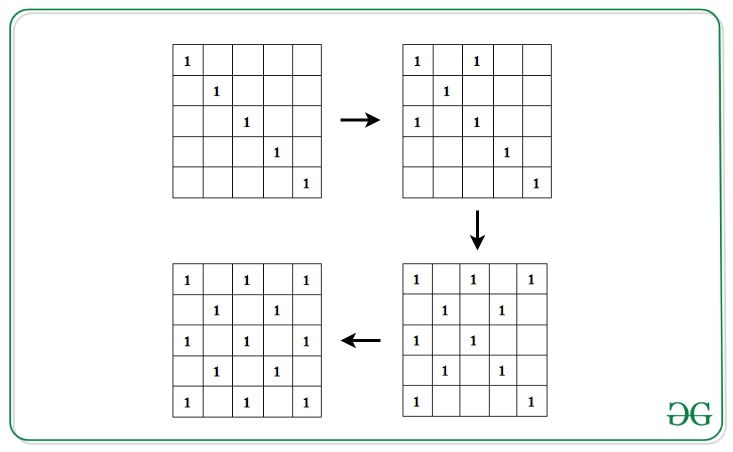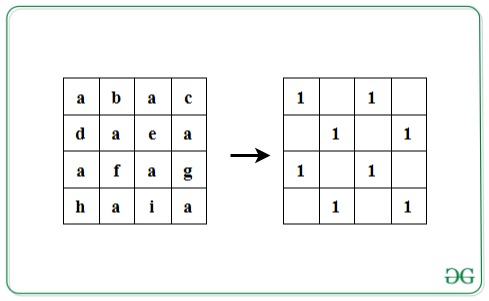GeeksforGeeks App
Open AppBrowser
Continue

# Check if given string satisfies the following conditions

Given a string S of length L, where L is a perfect square, the task is to check if the given string satisfies the following conditions:

• Insert the characters of the string into a square matrix A[][] of dimensions √L x √L in row-wise manner.
• Initialize another matrix M[][] with 0s. Fill the left diagonal with 1. Now, for 1 present in the left diagonal, fill its corresponding right diagonal with 1s.• Now, check if all the indices in matrix M[][] which contains 1, contains the same character in A[][].

If the condition is satisfied, print “Yes”. Otherwise, print “No”.

Examples:

Input: S = ”abacdaeaafaghaia”
Output: Yes
Explanation:Input: S = ”abacdaeabfaghaia”
Output: No

Approach: The idea is to traverse the matrix A[][] where its corresponding character in the matrix M[][] is 1. Follow the steps below to solve the problem:

• Calculate the dimensions of the matrix as N = √L.
• Iterate over the left diagonal by visiting each cell, A[i][i] where 1<= i<= N.
• For every element of the left diagonal at cell A[i][i], initialize variables x and y with i and traverse its corresponding right diagonal by visiting the character S[x*N + y] and S[y*N + x] and decrement x each time by 1 and increment y each time by 1 to move along the next cells in the right diagonals, while x is not smaller than 0 and y is smaller than N.
• If all characters are found to be same in the above step, print “Yes”. Otherwise, print “No” if any mismatch is found.

Below is the implementation of the above approach:

## C++

 `// C++ program for the above approach` `#include ``using` `namespace` `std;` `// Function to check if given string``// satisfies the given conditions``void` `isValid(string s)``{` `    ``// Dimensions``    ``int` `n = ``sqrt``(s.length());` `    ``char` `check = s;` `    ``// Left diagonal``    ``for` `(``int` `i = 0; i < n; i++) {``        ``int` `x = i, y = i;` `        ``// Right diagonal``        ``while` `(x >= 0 && y < n) {``            ``if` `(s[(n * x) + y] != check``                ``|| s[(n * y) + x] != check) {` `                ``// Conditions not satisfied``                ``cout << ``"No"` `<< endl;``                ``return``;``            ``}``            ``x--;``            ``y++;``        ``}``    ``}` `    ``// Print Yes``    ``cout << ``"Yes"` `<< endl;``}` `// Driver Code``int` `main()``{` `    ``// Given String``    ``string str = ``"abacdaeaafaghaia"``;` `    ``// Function call``    ``isValid(str);` `    ``return` `0;``}`

## Java

 `// Java program for the above approach``import` `java.util.*;``  ` `class` `GFG{``  ` `// Function to check if given string``// satisfies the given conditions``static` `void` `isValid(String s)``{``    ` `    ``// Dimensions``    ``int` `n = (``int``)Math.sqrt(s.length());``     ` `    ``char` `check = s.charAt(``0``);``     ` `    ``// Left diagonal``    ``for``(``int` `i = ``0``; i < n; i++)``    ``{``        ``int` `x = i, y = i;``        ` `        ``// Right diagonal``        ``while` `(x >= ``0` `&& y < n)``        ``{``            ``if` `(s.charAt((n * x) + y) != check ||``                ``s.charAt((n * y) + x) != check)``            ``{``                ` `                ``// Conditions not satisfied``                ``System.out.print(``"No"``);``                ``return``;``            ``}``            ``x--;``            ``y++;``        ``}``    ``}``    ` `    ``// Print Yes``    ``System.out.print(``"Yes"``);``}``  ` `// Driver Code``public` `static` `void` `main(String[] args)``{``    ` `    ``// Given String``    ``String str = ``"abacdaeaafaghaia"``;``    ` `    ``// Function call``    ``isValid(str);``}``}` `// This code is contributed by code_hunt`

## Python3

 `# Python3 program for the above approach``import` `math` `# Function to check if given string``# satisfies the given conditions``def` `isValid(s):``    ` `    ``# Dimensions``    ``n ``=` `int``(math.sqrt(``len``(s)))``    ``check ``=` `s[``0``]` `    ``# Left diagonal``    ``for` `i ``in` `range``(n):``        ``x ``=` `i``        ``y ``=` `i``        ` `        ``# Right diagonal``        ``while` `(x >``=` `0` `and` `y < n):``            ``if` `(s[n ``*` `x ``+` `y] !``=` `check ``or``                ``s[n ``*` `x ``+` `x] !``=` `check):``                    ` `                ``# Conditions not satisfied``                ``print``(``"No"``)``                ``return``                ` `            ``x ``-``=` `1``            ``y ``+``=` `1``            ` `    ``# Print Yes``    ``print``(``"Yes"``)``    ` `# Driver Code` `# Given String``str` `=` `"abacdaeaafaghaia"` `# Function call``isValid(``str``)` `# This code is contributed by avanitrachhadiya2155`

## C#

 `// C# program for the above approach ``using` `System;`` ` `class` `GFG{`` ` `// Function to check if given string``// satisfies the given conditions``static` `void` `isValid(``string` `s)``{``    ` `    ``// Dimensions``    ``int` `n = (``int``)Math.Sqrt(s.Length);``    ` `    ``char` `check = s;``    ` `    ``// Left diagonal``    ``for``(``int` `i = 0; i < n; i++)``    ``{``        ``int` `x = i, y = i;``        ` `        ``// Right diagonal``        ``while` `(x >= 0 && y < n)``        ``{``            ``if` `(s[(n * x) + y] != check ||``                ``s[(n * y) + x] != check)``            ``{``                ` `                ``// Conditions not satisfied``                ``Console.Write(``"No"``);``                ``return``;``            ``}``            ``x--;``            ``y++;``        ``}``    ``}`` ` `    ``// Print Yes``    ``Console.Write(``"Yes"``);``}` `// Driver code``public` `static` `void` `Main()``{``    ` `    ``// Given String``    ``string` `str = ``"abacdaeaafaghaia"``;`` ` `    ``// Function call``    ``isValid(str);``}``}` `// This code is contributed by sanjoy_62`

## Javascript

 ``

Output:

`Yes`

Time Complexity: O(L1/2) where L is the length of the given string.
Auxiliary Space: O(1) since constant space has been used

My Personal Notes arrow_drop_up